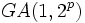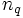# Order is product of Mersenne prime and one more implies normal Sylow subgroup

## Statement

Suppose$p$ is a prime number such that$2^p - 1$ is a Mersenne prime (?). Consider:$n = 2^p(2^p - 1)$.

Then, any group of order$n$ has a nontrivial Normal Sylow subgroup (?): either the$2$-Sylow subgroup is normal, or the$(2^p - 1)$-Sylow subgroup is normal. Thus,$G$ admits a Sylow tower (?), so$G$ is a Group having a Sylow tower (?).

## Examples

Some examples for small Mersenne primes are as follows:

•$p = 2, 2^p - 1 = 3, n = 12$: In a group of order$12$, either the$2$-Sylow subgroup or the$3$-Sylow subgroup is normal.
•$p = 3, 2^p - 1 = 7, n = 56$, In a group of order$56$, either the$2$-Sylow subgroup or the$7$-Sylow subgroup is normal.
•$p = 5, 2^p - 1 = 31, n = 992$: In a group of order$992$, either the$2$-Sylow subgroup or the$31$-Sylow subgroup is normal.

## Related facts

### Cannot pinpoint to any one prime

We can find groups of order$2^p(2^p - 1)$ where only the$2$-Sylow subgroup is normal, and we can find groups where only the other subgroup is normal. Here are examples of both:

• Only the$2$-Sylow subgroup is normal: Consider the general affine group$GA(1,2^p)$. This is the semidirect product of the additive group of a field of order$2^p$ with its multiplicative group. Here, only the$2$-Sylow subgroup is normal.
• Only the$(2^p - 1)$-Sylow subgroup is normal: Consider the direct product of the dihedral group of order$2(2^p - 1)$ and a cyclic group of order$2^{p-1}$. Here, only the$(2^p - 1)$-Sylow subgroup is normal.

## Proof

Given: A Mersenne prime$2^p - 1$, a group$G$ of order$2^p(2^p - 1)$.

To prove: Either the$2$-Sylow subgroup or the$(2^p - 1)$-Sylow subgroup of$G$ is normal.

Proof: Let$q = 2^p - 1$ and let$n_q$ and$n_2$ denote the number of$q$-Sylow subgroups and$2$-Sylow subgroups respectively. We have:$n_q \equiv 1 \mod q$.

Also, we have, since$n_q$ equals the index of the normalizer of a$q$-Sylow subgroup:$n_q | 2^p$

This forces either$n_q = 1$ or$n_q = 2^p$. If$n_q = 1$, we are done. Consider the case$n_q = 2^p$. Let$Q_1, Q_2, \dots , Q_{2^p}$ be all the$q$-Sylow subgroups. Since these are all groups of prime order, any two of them intersect trivially. Thus, the total number of non-identity elements in the subgroups is:$2^p(q - 1) = 2^p(2^p - 2) = n - 2^p$.

Thus, there are exactly$2^p$ elements that are not among the non-identity elements of$q$-Sylow subgroups. Note that any element in a$2$-Sylow subgroup cannot be among these non-identity elements of$q$-Sylow subgroups, so any element in a$2$-Sylow subgroup must be among these$2^p$ elements. This forces any$2$-Sylow subgroup to be contained in this set of$2^p$ elements. Since the order of a$2$-Sylow subgroup is$2^p$, this forces there to be a unique$2$-Sylow subgroup -- precisely those$2^p$ elements. This completes the proof.